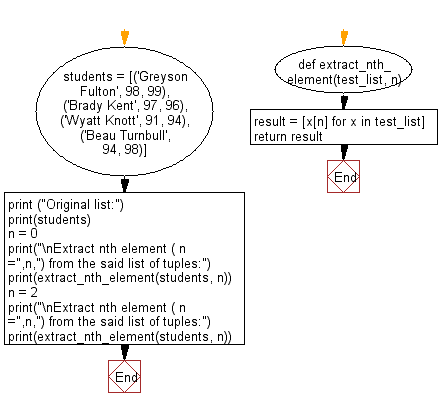﻿ Python: Extract the nth element from a given list of tuples - w3resource# Python: Extract the nth element from a given list of tuples

## Python List: Exercise - 114 with Solution

Write a Python program to extract the nth element from a given list of tuples.

Sample Solution:

Python Code:

``````def extract_nth_element(test_list, n):
result = [x[n] for x in test_list]
return result

students = [('Greyson Fulton', 98, 99), ('Brady Kent', 97, 96), ('Wyatt Knott', 91, 94), ('Beau Turnbull', 94, 98)]
print ("Original list:")
print(students)
n = 0
print("\nExtract nth element ( n =",n,") from the said list of tuples:")
print(extract_nth_element(students, n))

n = 2
print("\nExtract nth element ( n =",n,") from the said list of tuples:")
print(extract_nth_element(students, n))
```
```

Sample Output:

```Original list:
[('Greyson Fulton', 98, 99), ('Brady Kent', 97, 96), ('Wyatt Knott', 91, 94), ('Beau Turnbull', 94, 98)]

Extract nth element ( n = 0 ) from the said list of tuples:
['Greyson Fulton', 'Brady Kent', 'Wyatt Knott', 'Beau Turnbull']

Extract nth element ( n = 2 ) from the said list of tuples:
[99, 96, 94, 98]
```

Pictorial Presentation:Flowchart:## Visualize Python code execution:

The following tool visualize what the computer is doing step-by-step as it executes the said program:

Python Code Editor:

Have another way to solve this solution? Contribute your code (and comments) through Disqus.

What is the difficulty level of this exercise?

Test your Python skills with w3resource's quiz

﻿

## Python: Tips of the Day

```print(2_000_000)
```2000000